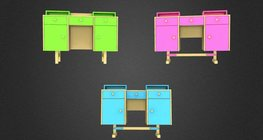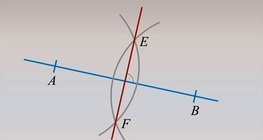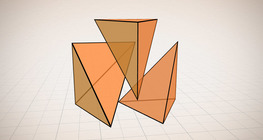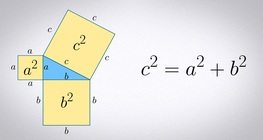Quantity: 0

Total: 0,00

0

# Robots

### Related extras#### Cube of cubes

An exercise about the regular hexahedron built from unit cubes to help deepen your...#### Sophie´s desk

A game about the different views of complex objects.#### Constructing the perpendicular bisector of a line segment

Let’s construct the perpendicular bisector of a 6-cm-long line segment.#### Volume of a tetrahedron

To calculate the volume of a tetrahedron we start by calculating the volume of a prism.#### Plotting parallel lines – Solution II

Let’s construct a rhombus such that one of its sides lies on line e and point P is one of the...#### Sphere

A sphere is the set of points which are all within the same distance from a given point...#### Grouping of cuboids

This animation demonstrates various types of cuboids through everyday objects.#### Weighing the Pythagorean theorem

In this experiment we provide proof of the well-known theorem of Pythagoras with a scale.

Added to your cart.